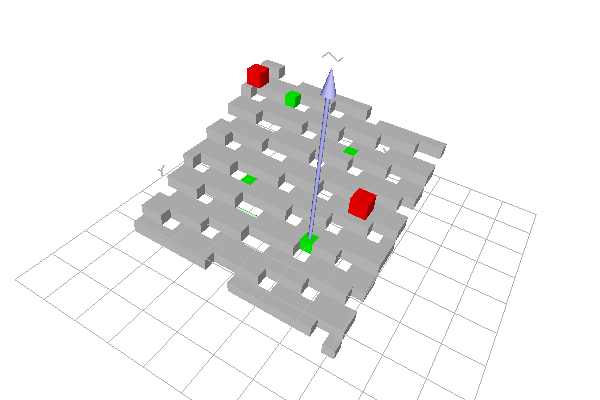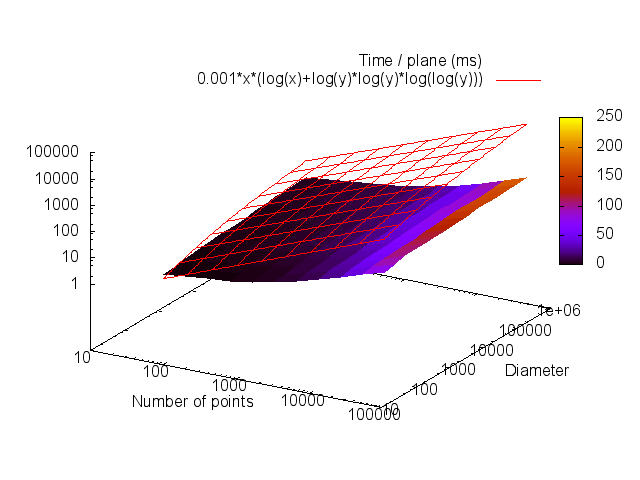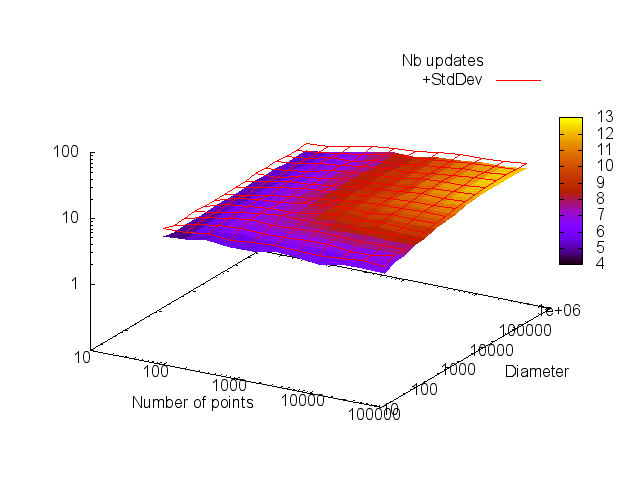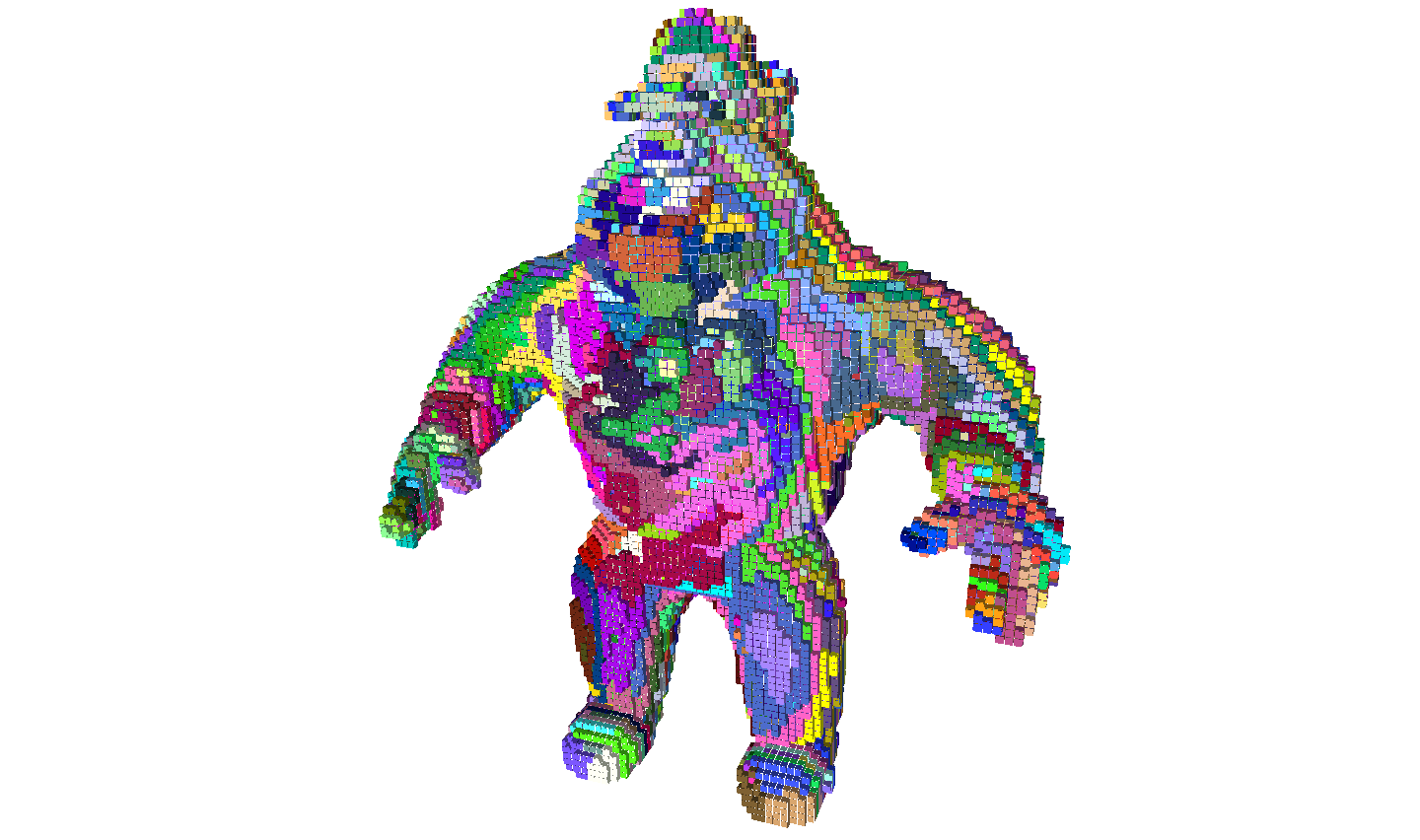DGtal  1.4.beta
Detailed explanation of COBA plane recognition algorithm
Author(s) of this documentation:
Jacques-Olivier Lachaud

Part of the Geometry package.

This module gathers classes and functions to recognize piece of naive planes, and more generally piece of planes with arbitrary rational axis-thickness. It is based on an implementation of the COBA Algorithm [Charrier, Buzer 1998 : ]. For the user, everything is gathered in classes COBANaivePlaneComputer and COBAGenericNaivePlaneComputer, which are additive primitive computers. File viewer3D-7-planes.cpp gives a very simple example of use-case. File greedy-plane-segmentation.cpp is a non trivial example that uses this plane recognition algorithm.

Related examples are viewer3D-7-planes.cpp, greedy-plane-segmentation.cpp, greedy-plane-segmentation-ex2.cpp.

# What planes are recognized by the COBA algorithm ?

The COBA algorithm recognizes planes defined with some equation $$\mu \le \vec{N} \cdot \vec{x} < \mu + \epsilon$$, where $$N_z$$ (for instance) is 1. This is ok for instance for recognizing naive planes defined by $$d \le ax+by+cz < d + \omega$$ where $$\omega = \max(a,b,c)$$. Assign indeed $$\mu = d / \omega, \vec{N} = (a,b,c) / \omega, \epsilon = 1$$. Extension to integer multiples or rational multiples of such planes is straightforward, and is hence provided.

The user thus specifies a main axis axis and a rational width (i.e. $$\epsilon$$ as widthNumerator / widthDenominator ) at initialization (see COBANaivePlaneComputer::init()).

The idea of the COBA algorithm is to transform the problem into a linear integer programming problem in the digital plane $$Z^2$$. If the main axis is z, then the algorithm looks for unknown variables $$(N_x,N_y)$$. Given a set of points $$(\vec{P}_i)$$, it computes (or maintains in the incremental form) the 2D space of solutions induced by these points. More precisely, each point induces two linear constraints $$\mu \le \vec{N} \cdot \vec{P}_i$$ and $$\vec{N} \cdot \vec{P}_i < \mu + \epsilon$$.

Instead of keeping this problem in $$R^2$$, the problem is cast in $$(hZ)^2$$, where the parameter h is the sampling step and is sufficiently small to capture a solution when there is one. When initializing the algorithm, the user must give the diameter of the set of points $$(\vec{P}_i)$$ (here largest vector in $$\infty$$ norm), see COBANaivePlaneComputer::init().

It is proven that if $$0 < h < 1/(2D^3)$$, where D is the diameter, then if there is a solution, there is a rational solution with denominator less than $$2D^3$$.

The COBA algorithm uses therefore a 2D lattice polytope (a convex polygon with vertices at integer coordinates), and class LatticePolytope2D, to maintain the set of rational solutions. Experimentally, even for a great number of points (<=100000), this convex set has generally fewer than 12 vertices (see Extracting plane characteristics).

A drawback of the COBA algorithm is that it does not provide the minimal characteristics of the recognized plane. This is due to the sampling method. In practice, the extracted normal is close to the minimal (integer) one.

Note
Another problem is that parameter $$\mu$$ is also unknown. To cope with that problem, a given solution (the centroid) is picked up and the maximal and minimal bounds are computed from it. If for a new point the bounds are too big, then a new direction is chosen by following the gradient of the induced constraints, and the algorithm optimizes iteratively the direction so as to get a direction with feasible bounds or no solutions. All this is hidden to the user, but explains the worst-case complexity.
You may have a look at Arithmetic package, more precisely at module Lattice polytopes in the digital plane ZxZ (convex polygons with vertices at integer coordinates) to see how lattice polytopes are represented.

# How to recognize a plane ?

The main class is COBANaivePlaneComputer. It is templated by the digital space TSpace, an arbitrary model of CSpace with dimension equal to 3, and by the internal integer type TInternalInteger, which should be an integral type with big enough precision to perform computations in the subsampled $$Z^2$$.

The most important inner types are (most of the others are redefinitions so that this object is a model of boost::ForwardContainer):

• Point: the type of point that will be checked in the algorithm. Defined as TSpace::Point.
• InternalInteger: the type of integer used in computations. Defined as TInternalInteger.
• PointSet: the type that defines the set of points stored in this object (for now, std::set<Point>).
• ConstIterator: the type of iterator to visit the set of points that composed the currently recognized plane.

You may instantiate a COBANaivePlaneComputer as follows:

#include "DGtal/geometry/surfaces/COBANaivePlaneComputer.h"
...
using namespace Z3i; // digital space is Z3
...
COBANaivePlaneComputer<Z3, int64_t> plane; // int64_t is enough for diameter 100
plane.init( 2, 100, 1, 1 ); // axis is z, diameter is 100, epsilon=1/1
Space Z3
Definition: StdDefs.h:145
boost::int64_t int64_t
signed 94-bit integer.
Definition: BasicTypes.h:74

Assuming you have a sequence of points stored in a container v, you may recognize if it is a piece of z-axis naive plane with the following piece of code:

bool isZNaivePlane( const vector<Point> & v )
{
COBANaivePlaneComputer<Z3, int64_t> plane; // int64_t is enough for diameter 500
plane.init( 2, 500, 1, 1 ); // axis is z, diameter is 500, epsilon=1/1
for ( vector<Point>::const_iterator it = v.begin(), itEnd = v.end();
it != itEnd; ++it )
if ( ! plane.extend( *it ) ) return false;
return true;
}

Therefore, the main methods for recognizing a plane are:

Note
Calling COBANaivePlaneComputer::isExtendable before COBANaivePlaneComputer::extend does not induce a speed up on the second method. You should prefer calling directly COBANaivePlaneComputer::extend() whenever you wish to extend the plane at this point.

You may also have a look at example viewer3D-7-planes.cpp.Piece of naive plane containing the four green points. There is no naive plane containing also any one of the red points.
Note
TInternalInteger specifies the type of integer used in internal computations. The type should be able to hold integers of order (2*D^3)^2 if D is the diameter of the set of digital points. In practice, diameter is limited to 20 for int32_t, diameter is approximately 500 for int64_t, and whatever with BigInteger/GMP integers. For huge diameters, the slow-down is polylogarithmic with the diameter.

# Extracting plane characteristics

You may obtain the current normal to the plane as a 3D vector over some real-value type (e.g. float, double) with the templated methods COBANaivePlaneComputer::getNormal (one component has norm 1) and COBANaivePlaneComputer::getUnitNormal (the 2-norm of the vector is 1).

You may obtain the upper and lower bounds of the scalar products with COBANaivePlaneComputer::getBounds.

Note
The COBA algorithm does not give you the minimal characteristics of the plane that contains all the input points. It provides you one feasible solution.

# Speed and computational complexity of COBA algorithm

It is not a trivial task to determine bounds on the computational complexity of this algorithm that depends only on the number of points N. Indeed, the determinant factor on the complexity is the number of times k that the plane parameters are updated. Another factor is the number of vertices v of the current convex set of solutions.

• The parameter k depends on the order of added point. It is related to the depth of bidimensional continued fraction of the plane normal (since each update corresponds to a refinement of the plane normal). A rule of thumb upper bound is some $$O(\log^2(D))$$, but experiments suggest a much lower bound.
• The parameter v seems mostly bounded in experiments. It is of course bounded by $$O(D^\frac{2}{3})$$, which is the 2D lattice polytope with the maximum number of sides in a D x D box. However, experiments suggest a more likely $$\log \log D$$.
• Note also that each time a new point is inserted, we check if it has already been added. With std::set, this implies a $$O(\log N)$$ cost.

Putting everything together gives some $$O(N \log N + k(v+N+\log(D))\log^2(D))$$. Neglecting v gives $$O( N (\log N + k \log^3 D ) )$$.

We have runned the following benchmark to estimate the average speed of COBA algorithm. For each experiment, 1000 naive planes were randomly chosen. Then, for an input diameter D, N points are randomly chosen in the parallelepiped D x D x D, such that they belong to the plane. We measure the time T to recognize these points as a naive plane (all calls to extend succeed). We also measure the number of updates k for this recognition. The following figures show the obtained results for a number of points varying from 50 to 100000 and a diameter varying from 50 to 1000000. It shows that the algorithm performs better in practice than the given upper bounds, even assuming $$k = \log \log D$$ and v constant.

Note
All computations were made with TInternalInteger set to BigInteger.Time (in ms) to recognize a naive plane as a function of the number of points N (x-axis) and the diameter (y-axis). Time is averaged over 1000 random recognitions.Number of updates (and deviation) when recognizing a naive plane, as a function of the number of points N (x-axis) and the diameter (y-axis). This number is averaged over 1000 random recognitions.

# Application to greedy segmentation into digital planes

Example greedy-plane-segmentation.cpp illustrates the use of COBANaivePlaneComputer to segment a digital surface into connected pieces of digital planes.

The surface will be define as some digital surface in a thresholded 3D image. The image is loaded here.

QApplication application(argc,argv);
DigitalSet set3d (image.domain());
SetFromImage<DigitalSet>::append<Image>(set3d, image, threshold,255);
ImageContainerBySTLVector< Domain, Value > Type
Definition: ImageSelector.h:78
static ImageContainer importVol(const std::string &filename, const Functor &aFunctor=Functor())
ImageContainerBySTLVector< Domain, Value > Image
Z2i::DigitalSet DigitalSet

Then the digital surface is built within this volume.

trace.beginBlock( "Set up digital surface." );
// We initializes the cellular grid space used for defining the
// digital surface.
KSpace ks;
bool ok = ks.init( set3d.domain().lowerBound(),
set3d.domain().upperBound(), true );
if ( ! ok ) std::cerr << "[KSpace.init] Failed." << std::endl;
MyDigitalSurfaceContainer* ptrSurfContainer =
new MyDigitalSurfaceContainer( ks, set3d, surfAdj );
MyDigitalSurface digSurf( ptrSurfContainer ); // acquired
void beginBlock(const std::string &keyword="")
double endBlock()
DigitalSurface< MyDigitalSurfaceContainer > MyDigitalSurface
DigitalSetBoundary< KSpace, DigitalSet > MyDigitalSurfaceContainer
Trace trace
Definition: Common.h:153

We define a few types. Note the COBANaivePlaneComputer and the structure SegmentedPlane, which will store information for each plane.

using namespace Z3i;
typedef COBANaivePlaneComputer<Z3,InternalInteger> NaivePlaneComputer;
// We choose the DigitalSetBoundary surface container in order to
// segment connected or unconnected surfaces.
typedef DigitalSetBoundary<KSpace,DigitalSet> MyDigitalSurfaceContainer;
typedef DigitalSurface<MyDigitalSurfaceContainer> MyDigitalSurface;
typedef SurfelSet::iterator SurfelSetIterator;
struct SegmentedPlane {
Color color;
};
SurfelSet::iterator SurfelSetIterator
COBANaivePlaneComputer< Z3, InternalInteger > NaivePlaneComputer
MyDigitalSurface::ConstIterator ConstIterator
MyDigitalSurface::SurfelSet SurfelSet
DGtal::int64_t InternalInteger
TriMesh::Vertex Vertex

We proceed to the segmentation itself. Note that we iterate over each surface element (surfel or vertex). Only vertices not processed can define the starting point of a new plane. Then a breadth-first traversal is initiated from this vertex and vertices are added to the plane as long as it is possible.

trace.beginBlock( "Segment into planes." );
std::set<Vertex> processedVertices;
std::vector<SegmentedPlane*> segmentedPlanes;
std::map<Vertex,SegmentedPlane*> v2plane;
Point p;
Dimension axis;
unsigned int j = 0;
unsigned int nb = digSurf.size();
for ( ConstIterator it = digSurf.begin(), itE= digSurf.end(); it != itE; ++it )
{
if ( ( (++j) % 50 == 0 ) || ( j == nb ) ) trace.progressBar( j, nb );
Vertex v = *it;
if ( processedVertices.find( v ) != processedVertices.end() ) // already in set
continue; // process to next vertex
SegmentedPlane* ptrSegment = new SegmentedPlane;
segmentedPlanes.push_back( ptrSegment ); // to delete them afterwards.
axis = ks.sOrthDir( v );
ptrSegment->plane.init( axis, 500, widthNum, widthDen );
// The visitor takes care of all the breadth-first traversal.
Visitor visitor( digSurf, v );
while ( ! visitor.finished() )
{
Visitor::Node node = visitor.current();
v = node.first;
if ( processedVertices.find( v ) == processedVertices.end() )
{ // Vertex is not in processedVertices
axis = ks.sOrthDir( v );
p = ks.sCoords( ks.sDirectIncident( v, axis ) );
bool isExtended = ptrSegment->plane.extend( p );
if ( isExtended )
{ // surfel is in plane.
processedVertices.insert( v );
v2plane[ v ] = ptrSegment;
visitor.expand();
}
else // surfel is not in plane and should not be used in the visit.
visitor.ignore();
}
else // surfel is already in some plane.
visitor.ignore();
}
// Assign random color for each plane.
ptrSegment->color = Color( rand() % 256, rand() % 256, rand() % 256, 255 );
}
void progressBar(const double currentValue, const double maximalValue)
DGtal::uint32_t Dimension
Definition: Common.h:136
MyPointD Point
Definition: testClone2.cpp:383

We display it using 3D viewers.

Viewer3D<> viewer( ks );
viewer.show();
for ( std::map<Vertex,SegmentedPlane*>::const_iterator
it = v2plane.begin(), itE = v2plane.end();
it != itE; ++it )
{
viewer << CustomColors3D( it->second->color, it->second->color );
viewer << ks.unsigns( it->first );
}
viewer << Viewer3D<>::updateDisplay;Greedy segmentation of Al capone into naive planes.
Note
This approach to plane segmentation is very naive. Because there are favored axes when iterating (vertices are not randomly picked up), this technique has indeed the drawback of favoring "slice planes", which are not very useful.
Exercice 1. Randomize the greedy plane recognition. The simplest approach is first to put all vertices into a vector, then suffle it with STL algorithms. And then iterate over this shuffled set.
Exercice 2. Enhance the polyhedrization by selecting first the vertices that induces the biggest planes. This is much slower than above, but will give nicer results. For each vertex, computes its best plane by breadth-first traversal. Stores the obtained size (or better moments). Do that for each vertex independently. Put them in a priority queue, the first to be popped should be the ones with the biggest size. The remaining of the algorithm is unchanged.

# What if you do not know the main axis beforehands ?

In this case, you should use the class COBAGenericNaivePlaneComputer. You use it similarly to COBANaivePlaneComputer, but you do not need to specify a main axis when calling COBAGenericNaivePlaneComputer::init().

#include "DGtal/geometry/surfaces/COBAGenericNaivePlaneComputer.h"
...
using namespace Z3i; // digital space is Z3
...
COBAGenericNaivePlaneComputer<Z3, int64_t> plane; // int64_t is enough for diameter 100
plane.init( 100, 1, 1 ); // diameter is 100, epsilon=1/1

Then, you may use methods COBAGenericNaivePlaneComputer::clear(), COBAGenericNaivePlaneComputer::extend(), COBAGenericNaivePlaneComputer::isExtendable(), etc, similarly as for class COBANaivePlaneComputer. The advantage is that the object detects progressively what is the correct main axis. You may know what is a correct main axis by calling COBAGenericNaivePlaneComputer::active().

Note
The principle of COBAGenericNaivePlaneComputer is to have three instances of COBANaivePlaneComputer at the beginning, one per possible axis. When extending the object by adding points, all active instances are extended. If any one of the active instance fails, it is removed from the active instances. It is guaranteed that if there is a naive plane (of specified width) that contains the given set of points, then the object COBAGenericNaivePlaneComputer will have at least one active COBANaivePlaneComputer instance. COBAGenericNaivePlaneComputer is thus a correct recognizer of arbitrary pieces of naive planes.